Math Play

From the time I was a teenager, I am fascinated by mathematics. Starting when I was 12 years old, math became more and more a hobby of mine. I experimented with it in all kinds of ways.

You know: it's so strange. Lots of people find culture very important. They speak highly of Michelangelo, Johann Wolfgang von Goethe and Krzysztof Penderecki; in short, they value their own cultural level highly.

At the same time, many of them are openly proud that they understand little or nothing about mathematics. In so doing, they deny that mathematics is an equally high-profile part of our ancient and contemporary culture.

It would go too far to state that it is barbaric, not to love mathematics. But I dare say that it is barbaric to disdain mathematics, like many do. But perhaps I can show you on this page, that math can be highly cultural, challenging the intellect and the emotions alike.

My motto in math: The fun is in the solving, not in the solution. I'll show you why, if you allow me.So, if you really want to have fun, then read the problems on this page and solve them yourself; next compare your solutions with mine. Or if yoy don't, then at least read about my adventures in math.

On this page: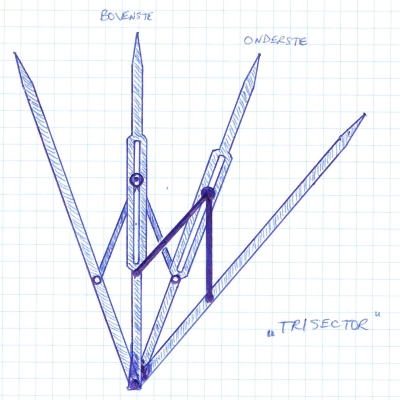2. Magic Squares

3. Super Spheres

4. The Third Degree Polynomial Equation  (and the Fourth)

5. Rubik's Cube

6. The three classical geometry problems

7. Overwhelming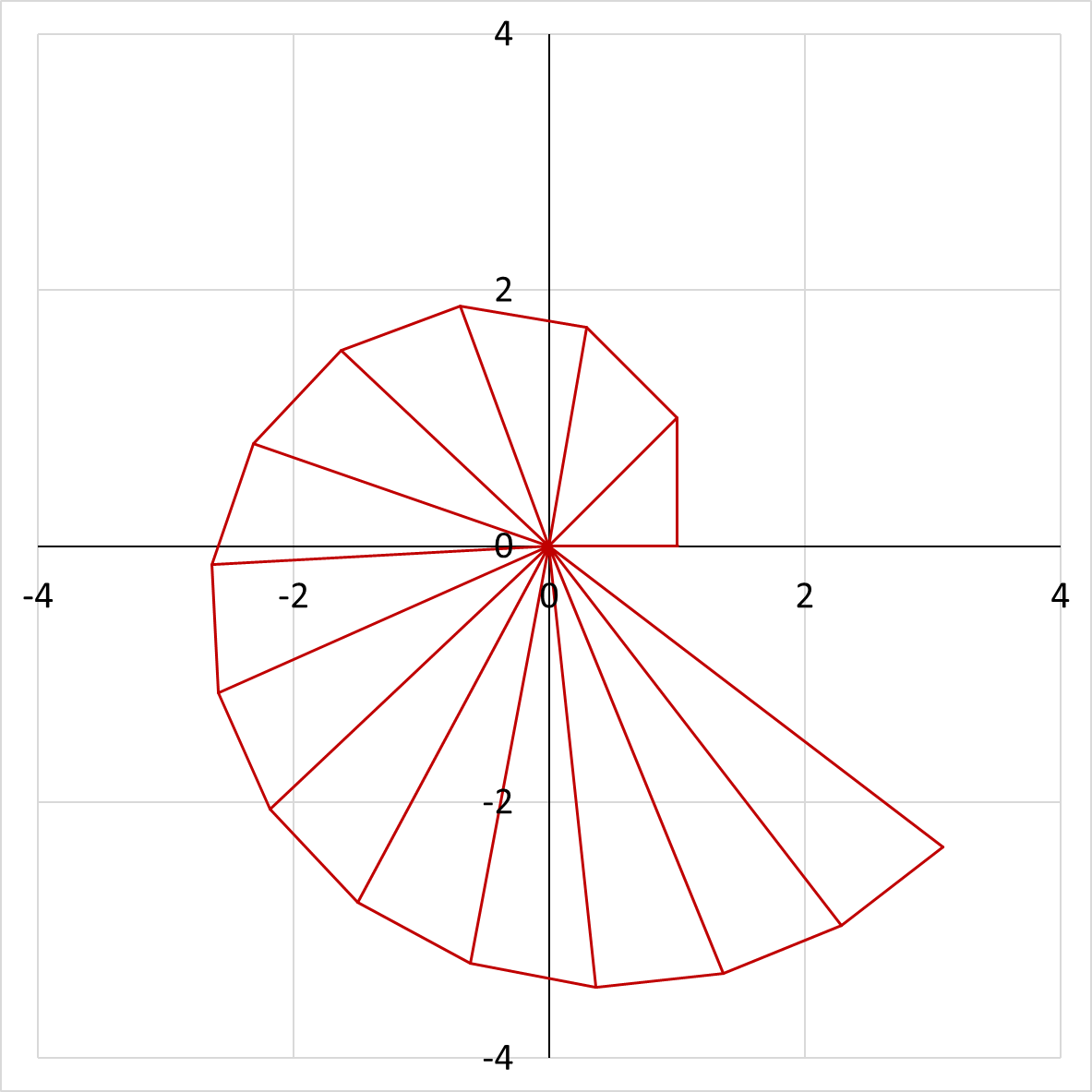1. Pythagorean spiral

At a certain moment - I guess I was 15 years old or so - I thought it would be interesting to draw a whole series of rectangular triangles, in the way I repeated here in the image on the right. When I did that the first time, I had to do it by hand on a large sheet of paper, to which I glued more sheets when the figure got bigger and bigger. Right now, when I add this to my website, the computer helps; I just did the calculations using Excel, which shows the results in a graph.

I did it as follows. First I drew a horizontal line to the right with a length of 1. From the right end I drew a line straight up, also with a length of 1. I connected the two separate endpoints and now had a rectangular triangle, of which Pythagoras teaches us that the hypotenuse has a length of √2.

Perpendicular to the hypotenuse, I drew another line with length 1. I connected the end point with the original beginning, so I got a second triangle, now with a hypotenuse with length √3.

The third triangle had a hypotenuse with length √4, or: exactly 2.

I continued in this way, so that a spiral came into being which was made up entirely of rectangular triangles. I kept that up for a long time, so that eventually a spiral appeared with several complete windings: see the large figure.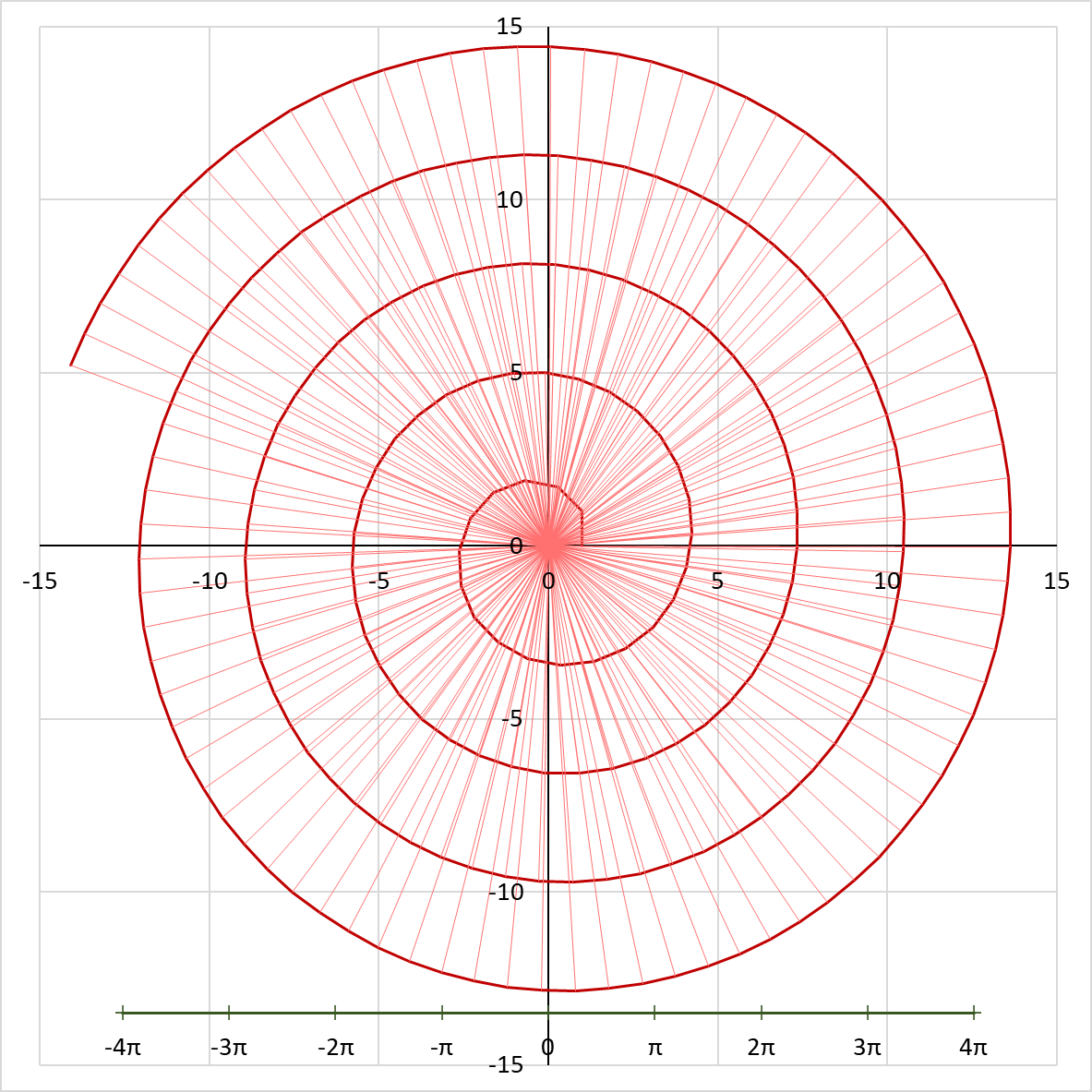The result yielded two fascinating surprises. To start with: the successive windings of the spiral were bigger and bigger, that goes without saying. But I expected that the distances between the windings would also increase. And that is not the case! The distance between the windings turned out to be constant. This obviously raised the question of how large that distance is.

You cannot answer that question exactly, because the spiral is not a fluent line but consists of straight pieces. But if you take about the average of those, then the second surprise appears. That distance is: π ! The famous circle number, approximately equal to 3.14. This was an astonishing discovery. The mathematics teacher in high school, whom I showed the result, agreed enthusiastically with me.

This phenomenon yielded an additional conclusion, free of charge. I knew by then, that it had been proven that you cannot construct π exactly with geometrical means. With that fact I was able to apply a proof by contradiction. Look: you might expect that, if you construct enough spirals in the way I have thought of, one day you would be able to find two triangles of which the hypothenuses coincide exactly with each other. But if so, I would have constructed π (or a multiple of it, if not adjacent spirals), precisely along that path. From the fact that it has already been demonstrated that this is not possible, it is indisputable that such exactly coinciding triangles do not appear, even if you would continue to draw triangles indefinitely.

I have never found this 'Pythagorean Spiral' anywhere in mathematical literature, but I have no doubt that it will have been found somewhere. I do not have the illusion that I could discover something really new with my mathematical Spiral, or with any other of my math recreations. That wasn't my ambition either: I just wanted to have nice adventures and solve exciting mathematical puzzles, even if I didn't do it first.

2. Magic squares

One beautiful day, I began to wonder how to make magic squares of any size. You know: squares of squares, n x n large, in which you fill in the natural numbers 1 to n², such that all rows, all columns and both main diagonals all add up to the same value. A recipe for any size: that seemed like a nice puzzle to me.
The first few sizes are simple. As a start there is of course the trivial square n=1: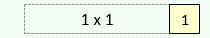Then n=2, which is even simpler, because it can't be done at all. No matter how you fill in the digits 1 to 4, you'll never meet the conditions. It is not difficult to check, because there are exactly 4! = 24 ways to fill in the digits, and when you take rotation and mirror symmetry into account, there are only 3. And all three don't work.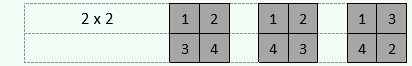From n=3 it started to get interesting. Soon it turned out that squares with an odd n are fairly easy to make. The example with n=13 shows how the recipe works.

First, fill in the number 1, in the box right below the middle.

Now go 1 to the left and 1 to the bottom. Take the value you just filled in, add n, and fill that in. In the example: 1 + 13 = 14. Repeat that, and fill in successively: 27, 40, etc. When you reach the bottom of the square, jump to the top. Ditto, when you arrive on the left, jump to the far right. The last number you enter is n² - n + 1, in the example 157.

Now take successively each of the just filled in numbers as a starting point. From that number go 1 to the right and 1 to the bottom. Take the number you just left, add 1, and fill in the answer. Continue like this. Again you jump over the edge when necessary: from right to left and from bottom to top. Repeat that until you're done, and the whole square will be filled neatly.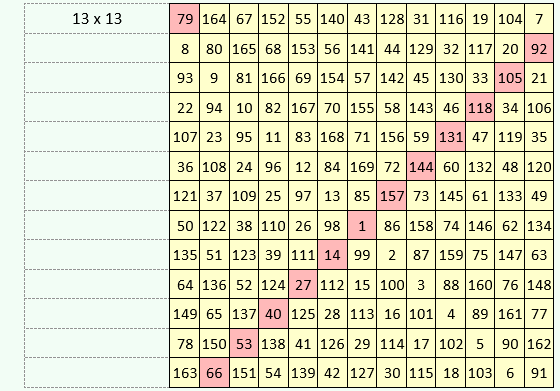If n is even, it runs differently. How, that depends on whether n is double-even, i.e. divisible by 4, or not. The 4-fold is very easy. Just look at n=12.
You make a checkered pattern of 2 x 2 squares. In the example I colored those yellow and green. Along the edge, the squares are only half, and in the corners only a quarter of 4 squares.
Now simply enter the numbers from 1 to n² in the yellow boxes, starting at the top left and writing from left to right and then from top to bottom, just like normal (western) text. Continu counting in the green boxes, but don't fill in the numbers.
Then do the opposite. Start just right at the bottom. Travel to the left and fill in the green boxes, again with the numbers 1 through n², this time counting through the yellow boxes but not filling them in.
And voilà, that's how you get the magic square.But now the n-values that are even but not double-even! They are a bit more difficult.
With n=12, I just used two counting systems: one from the top left (yellow), the other from the bottom right (green). For n=14, and the other even-but-not double-even sizes, you even use four counting systems: the ones mentioned just now, plus one starting from the bottom left (blue) and one from the top right (red).

To fill in everything, first fill in the 'W'-shaped blue boxes, followed by the 'tilted W'-shaped red boxes. Then fill the rest with a diamond pattern of yellow and green, making sure that the pattern is discontinuous along the horizontal and vertical centerlines.

This approach can be done consistently for n=6, 10, 14, 18, etcetera.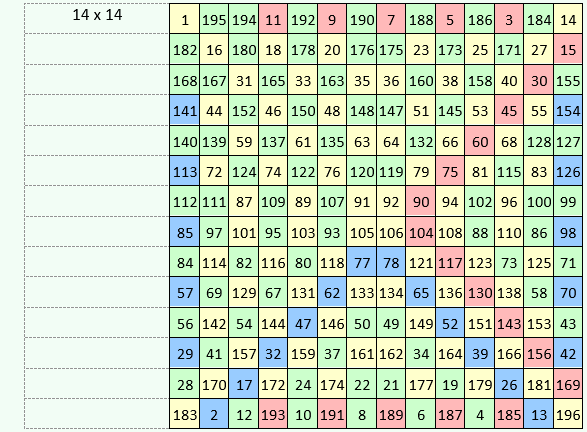This solves the problem of the magic squares: you can produce one for any size, except n=2. That does not mean that you then have all magic squares, because it is not difficult to make many more.

An example? Take one of the squares shown above. Swap row 1 and row 2. Then also swap column 1 and column 2. The result is guaranteed to be another magic square.3. Super spheres

A while after my adventure with the magic squares, I had another fun puzzle.

You have circles and spheres. A circle in a two-dimensional space (a flat surface), is the equivalent of a sphere in a three-dimensional one: both consist of the set of all points that have a distance of R (the Radius) to a given center.

The measure for the 'volume' of a circle is its surface area, which is πR², as you can find in any mathematics textbook. For a sphere, the volume has a value of 4/3 πR³. That's all well-known.

First: down to the one-dimensional world, i.e.: a line segment. This is simple, because the set of points with a distance R to a given center consists of exactly two separate points, one to the left and one to the right of the center. The 'volume' here is the length in between, which is 2R.

Let's go up, now. How to calculate the 'volume' of a four-dimensional sphere? A ten-dimensional one? Nice puzzle. After some thought I concluded that the correct formula for the calculation is: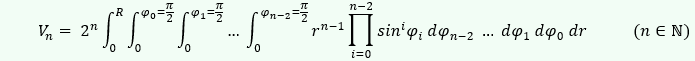This resulted in the following table, with some remarkable findings. For example, that for every two extra dimensions one extra π creeps into the formula. Strange, isn't it?

Anyway, the results for even dimensions look very different from their odd colleagues. That is why I split the table in two.Was there any logic in that? In other words, could you draw up a few general formulas that would allow you to easily find any volume? Sure: you can easily discover them by looking in the table at dimensions 13 and 14: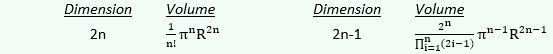For both even and odd dimensions, the iterative formula applies: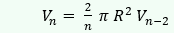When you inversely apply this iterative formula to determine V0 from V2, you get the value 1, which is why I assigned this value by definition and filled it out in the table above. A strange value maybe, but not stranger than another, generally accepted definition:
0! = 1.

4. The Third Degree Polynomial Equation  (and the Fourth)

A new question came to me that I couldn't let go.

There is a well-known class of equations in math that often need to be solved for purposes in real life. This is the class:

General  1st degree polynomial equation: ax + b =0

General 2nd degree polynomial equation: ax2 + bx + c = 0

General 3rd degree polynomial equation: ax3 + bx2 + cx + d = 0

General 4th degree polynomial equation: ax4 + bx3 + cx2 + dx + e = 0

Etc.

The First and Second Degree
Now, the solution to the general 1st degree equation is very simple. The 2nd degree equation is quite easy, too: the solution is called the abc formula, and it is taught in the math classes in high school all over the world. Below, on the left, you see the 1st degree solution. On the right the 2nd degree solution is shown, often called the abc formula, which in general renders two different solutions: x1,2, which differ thanks to the 'Discriminant' that, in the formula, is added or subtracted, creating two different solutions.The Third Degree
This is all very ordinary stuff. But how about the 3rd degree equation? This kept me busy for several months. Of course, I would certainly not be the first one to solve this problem, I was well aware of that. But nevertheless I found it a very challenging problem that I absolutely needed to solve.

I tried hard. In my notebook (a paper one, of course; the present-day notebooks are laptop computers, but when I dedicated my time to this problem, they did not exist for several decades yet). Here are three pages from my notebook: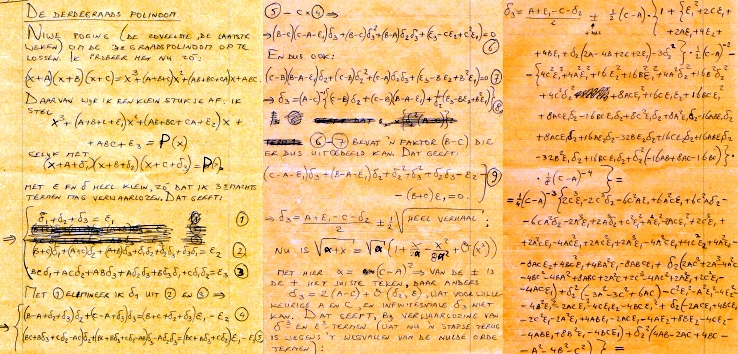I tried many ways to find a solution, but all my attempts were in vain. Until finally, one beautiful morning, I wrote in my notebook: "The true problem in the third-degree polynomial". That proved to be correct. In my latest analysis, I realized: the ± in the abc formula stands for ±1, i.e. for the two solutions of the simplest second-degree equation:
x² = 1.

If I wanted to solve a third-degree equation, I had to do something with the three solutions of the simplest third-degree equation:
x³ = 1.

I necessarily had to find these three solutions in the complex plane, as the picture below shows.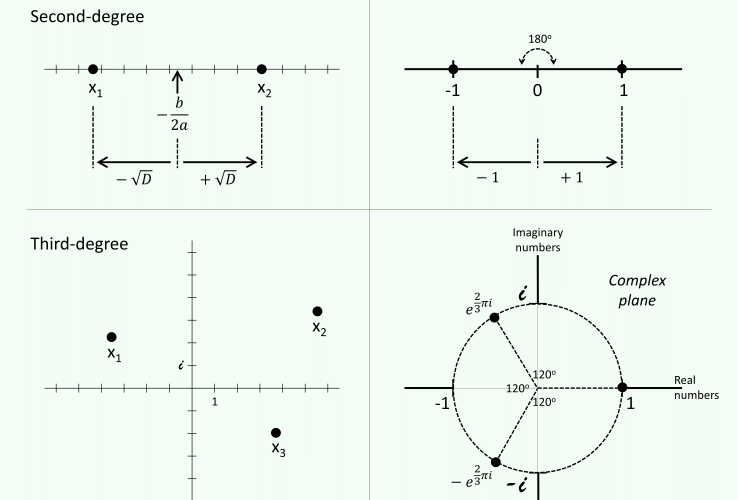In other words, instead of ±1, I needed three numbers: 1,   eπi   and   e-πi . (Of course, i is equal to the square root of -1). Together, I called them k1,2,3. They are the three values shown next to the unit circle in the figure on the right; the two complex numbers are equal to -½ ± ½i√3.

Starting from this general idea, I was able to solve the problem and formulate a general solution (shown in my notebook on page 410 etc.), in which I made use of two different Discriminants:You could call this solution, following the example of the second-degree solution, the abcd formula.

I made many checks by inserting a range of different numbers and checking the solutions. This was a hell of a job, as I had to do it all by hand & head, without computer or calculator: something a modern-day person can hardly imagine. Anyway: the checks scored 100% correct.

The Fourth Degree
Of course I was delighted. But not yet satisfied: now I needed to see if my approach also worked for the 4th degree equation. This was an enormous job, much bigger than the third degree problem; but luckily I now knew how to approach it.

After a while, I found the following abcde formula (described in my notebook on page 419 etc.), making use of a layered set of Discriminants:There is one unfortunate aspect of this result: the ± in the formula for D1,2,3. In some cases (e.g. x1,2,3,4 = 0, -1, -1, -1 and -2, -1, 0, 1) this appears to have to be a +, while in some other cases (e.g. x1,2,3,4 = -1, 0, 0, 0 and 2, 1, 0, 1) it has to be a –. This is an ambiguousness for which I did not find a solution, and after a while I stopped looking for one, as I realized that this is not a major issue: every fourth degree equation is still solvable, because: if you end up with two sets of solutions, you can simply check which is the right one by substituting the two sets of values into the original equation.

Fifth and higher
I made no attempt to solve the Fifth or higher Degree equation, as I knew from literature that it has been proven that a general solution for these equations is impossible - although I never saw this proof. Maybe I will one time try to reproduce that proof myself.

Nowadays, if I would be looking for a general solution for math problems like these, I would find them within a few seconds, simply by googling them: probably, Wikipedia will have them. But what fun is there in being presented for free with such solutions? The internet brings a lot of pleasure and knowledge; but it is also a big spoiler. Because:
The fun is in the solving, not in the solution.5. Rubik's Cube

The fun is in the solving, not in the solution. This is very much the truth for another kind of problem that presented itself.

(Photo by Lars Karlsson (Keqs) on Wikimedia.)

When Rubik's cube first appeared in stores, it didn't take long before all kinds of people - mainly boys, I think - turned it into a sport to solve the cube as quickly as possible. Some could do it in less than half a minute.

But of course, that wasn't solving it at all, what they did.

What they did was unravel the cube. From a chaotic state to the most orderly state. They used standard recipes they learned from others, or from a booklet. Nothing creative, just applying algorithms and heuristics learned by heart.

No, solving the cube was something completely different. Namely: designing your own algorithms and heuristics, without any help, that allow you to transform the cube from any initial state to the most ordered state. This was a fantastic puzzle, which I threw myself into in 1981, when the cube came on the market. I did it as follows.

Step 1: definitions
The first step was to give names so that I could write down my actions. I chose for instance:

Layers of the cube in capital letters:

• B = above (Dutch: "Boven"), M = middle (horizontal), O = below ("Onder"),
• V = front (Dutch: "Voor"), F ("Face") = center (parallel to V), A = rear ("Achter"),
• L = left, P ("Profile") = center (parallel to L), R = right.

Bricks in lowercase:

• x = center block of a plane, e.g. v = center block of V
• xx = rib cube, at the center of a rib, e.g. vr = block at the edge of V and R
• xxx = corner block, e.g. vrb = block at the corner of V, R and B.
• ⊞ = 5 blocks in one layer: the whole layer except the corner blocks.
• ⊠ = 5 blocks in one layer: the center and the four corner blocks, the inverse of ⊞.
• π = the place of a block (regardless of its position)
• ω = the position of a block (regardless of location)

Cube states:

• ψ = 'state', the state in which the cube is at a given moment.
• ψ0 = initial state, the state in which you buy the cube, with the highest possible order: 1 color per plane.

Operators, i.e. operations you apply to the cube, with the same capital letters as the plane names.
For example:

• X = any operator.
• B = rotate the top surface a quarter turn clockwise.
• BL = First rotate the upper surface, then the left surface, both a quarter turn clockwise.
• B2 = BB = rotate the upper surface half a turn (= 2x a quarter turn) clockwise (or against it, that amounts to the same thing).
• B† = the inverse of B: Turn the upper surface a quarter turn counterclockwise.
• I = the 'identity', i.e. the zero operator: do nothing; or do such a series of actions that the end result is that you return to the state you started with.
• QX = Rotation of the whole cube, a quarter turn clockwise as seen from plane X, where the state (ψ) does not change and where plane X does not change location. For example:
• QL results in: L → L, R → B → V → O → A → B (i.e. L and R remain in place, but B occurs, V comes under, etc.)

Effects of series operators, such as:

• xx½ = rib cube xx rotates half a turn
• xxx⅓ = corner block xxx rotates 120o clockwise
• xxx-⅓ = idem, counterclockwise

Step 2: Discovery of laws and useful actions
With that kind of notation I could start experimenting. Perform small series of actions on the cube, see what happens, and then quickly return the cube to ψ0, the virgin state. Prevent the cube from ending up in chaos from which I cannot return to the initial state!

Propositions
I found simple propositions, such as:

- If X is a single operator (i.e.) rotating one plane, then:

• X2 = X†2
• X3 = X†
• X4 = I

- Also:

• (XY)† = Y†X†
• XI = IX = X
• (X†)† = X

Operators
I discovered useful sets of operators and gave them special names, such as:

 Operator         X1 = (R2B2) R (R2B2)†         X2 = (R2B2) R2 (R2B2)† R2         ...         X6 = (RALV) B (RALV)† B† M         X7 = X1 B2 X1         ... (Etc.) Effect ar → bl → vr → br → ar.   (I.e. cyclic: moves ar to bl, bl to vr, vr to br, br to ar.) ba ↔ bv  and  ra ↔ rv.   ba½  and  bl½ (B⊞)2  (I.e.: only the 4 rib cubes of B make twice a quarter turn).

Conservation laws
Of course I found a series of conservation laws. They made the cube to look more and more like a mathematical or physical system. Very intriguing! The cube was a small cosmos with its own laws of nature.

Conservation laws were illustrated by operators like X2, where you can see that you can't change ba and bv with impunity: you pay a price in the form of an exchange of ra and rv at the same time. Or X6: Do you want to reverse ba? Fine, provided that bl also tilts at the same time. Nothing is free!

This also proved that there are 'forbidden states' of the cube, such as a state where ba and bv are swapped and nothing else. Such a state is inaccessible from ψ0.

This also means that if you would take the cube apart (literally physically), and put the parts back together again in such a way that such a 'forbidden state' would arise, you would never be able to bring the cube back into the initial state ψ0 without demolishing the cube again.

Step 3: The Solution
The operators have the form of algorithms: fixed series of operations with fixed effects. But solving the cube problem, i.e. reaching ψ0 from any state ψ, can never take the form of an algorithm.

This is a consequence of the randomness of the chaotic starting state. Starting from that state, you can only give a set of instructions, looking closely at what you have in your hands, to put parts of the cube in order, and eventually the cube as a whole.

So, these prescriptions are heuristics: guidelines.

First, I designed a number of basic heuristics. Using these, I was able to formulate a composite heuristic with which you can always solve the cube.

Basic heuristics

 Basic Heuristic         H(M⊠)         H(B⊠π)         H(B⊠ω)         ... (Etc.) Effect Use X1 and X2 (bringing the 4 corner blocks of M in order) Use X3, X11, X12 (to fix the location of the corner blocks of B) Use X4 (to adjust the orientation of the corner blocks of B)

Solution heuristic
And the solution is:

H(Q) = H(O) H(O) H(M) H(½M) H(B⊠ π) H(B⊠ ω) H(½M + B)

That's it! Guaranteed to work.

When I had reached this point, I experimented a bit more to see if I could make the solution even more efficient or elegant. I succeeded a number of times, but at a certain point that of course didn't work.

After this, the problem was completed and the cube was put away. It was a beautiful puzzle! But after finding the solution it was just like a completed crossword puzzle:

Finished.

6. The three classical geometry problems

I know quite well that you have to solve all geometric problems in the traditional way, using only ruler, pen and compass, including the three 'classical geometric problems'. And I also know that these three are then insoluble: that has been proven.

But I thought it would be nice to think how you could solve them if you could invent and apply new tools. By cheating, I admit it - but just a little.

a. Doubling the cube

Assignment: create a cube that has twice the volume of a given cube.

This is impossible, because the larger cube must have a rib that is ∛2 times that of the smaller one. And that length cannot be constructed by classical means. But with the doubler© it definitely can: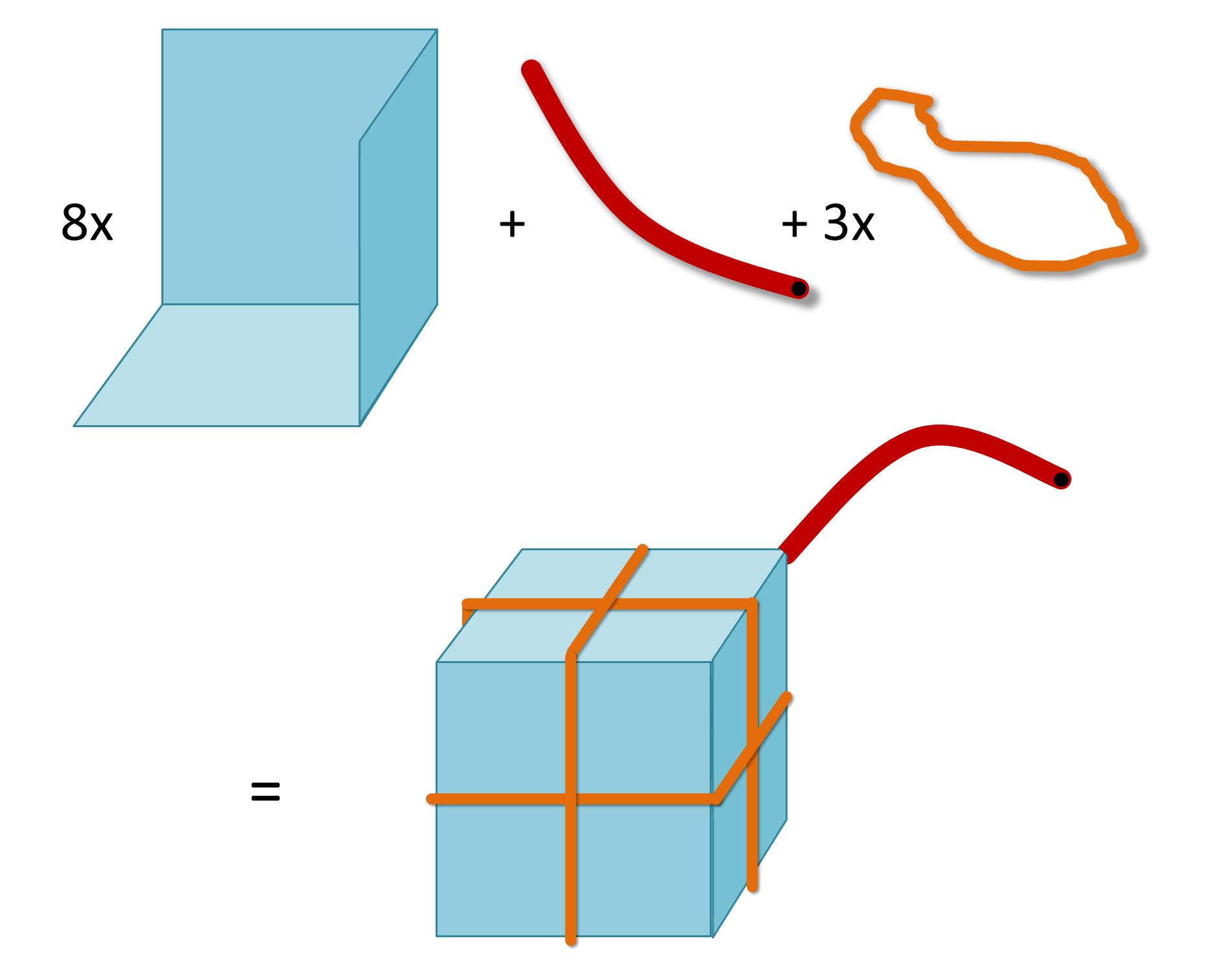First you make eight angular shapes, each consisting of three square planes whose side is equal to the rib of the given cube. Put them all together, tied together with three identical rubber bands. Now you have a closed cube.

At one of the corners, make an infinitesimal (or at least negligible) hole, to which you connect a tube. Fill the cube with water. Let that water out again and keep it carefully. Fill the cube again with water.

Now press the water from the first time into the cube, causing it to grow, while the rubber bands stretch. Since the resilience in the elastic bands increases proportionally to the stretching, this guarantees that all elastic bands stretch equally: in total this produces the minimum resilience, and thus the minimum energy input.

The result is another cube, with twice the volume of the first one.

b. Trisecting the angle

Assignment: divide any given angle into three equal parts.

With compass and ruler you can't do that. But with the trisector© you can! I show you the picture of my invention, taken directly from my personal Notebook, page 663 (July 5, 1984):Let the outer two points coincide with the angle to be divided. The inner two divide it neatly into three.

c. Squaring the circle

Assignment: Create a square with the same surface area as a given circle.

This is not possible either, this time because it requires the number π to be constructed with compasses and rulers.

Stretch a non-elastic string around the given circle and tie it tight. Now put it loosely around the doubler©, parallel to one of the three elastic bands. Pump water into the cube so it swells, until the string is pulled tight. Each of the six cube planes is now equal to the requested square. There you are!

7. Overwhelming

Addition is accelerated counting. Thus, 5 + 4 equals 5 + 1 + 1 + 1 + 1, and the answer is the fourth successor of 5, namely 9. That is no nonsense, I am not making it up: that is how it is really defined in mathematics.

Multiplication is accelerated addition. For example, 4 x 5 equals 5 + 5 + 5 + 5, or if you prefer, 4 + 4 + 4 + 4 + 4. This is much faster than addition: the result is 20.

Exponentiation is accelerated multiplication. For example, 54 equals 5 x 5 x 5 x 5. That's much faster again; the result is 625.

And if you want to go a step further? For example, how could you speed upSpontaneously, I called this operation 'overwhelming', when I thought about it in 1982. That's a fitting name, because this process goes very fast: the result is 53125, which is

1911012597945477520356404559703964599198081048990094337139512789246520530242615803012059386519739850265586440155794462235359212788673806972288410146915986602087961896757195701839281660338047611225975533626101001482651123413147768252411493094447176965282756285196737514395357542479093219206641883011787169122552421070050709064674382870851449950256586194461543183511379849133691779928127433840431549236855526783596374102105331546031353725325748636909159778690328266459182983815230286936572873691422648131291743762136325730321645282979486862576245362218017673224940567642819360078720713837072355305446356153946401185348493792719514594505508232749221605848912910945189959948686199543147666938013037176163592594479746164220050885079469804487133205133160739134230540198872570038329801246050197013467397175909027389493923817315786996845899794781068042822436093783946335265422815704302832442385515082316490967285712171708123232790481817268327510112746782317410985888683708522000711733492253913322300756147180429007527677793352306200618286012455254243061006894805446584704820650982664319360960388736258510747074340636286976576702699258649953557976318173902550891331223294743930343956161328334072831663498258145226862004307799084688103804187368324800903873596212919633602583120781673673742533322879296907205490595621406888825991244581842379597863476484315673760923625090371511798941424262270220066286486867868710182980872802560693101949280830825044198424796792058908817112327192301455582916746795197430548026404646854002733993860798594465961501752586965811447568510041568687730903712482535343839285397598749458497050038225012489284001826590056251286187629938044407340142347062055785305325034918189589707199305662188512963187501743535960282201038211616048545121039313312256332260766436236688296850208839496142830484739113991669622649948563685234712873294796680884509405893951104650944137909502276545653133018670633521323028460519434381399810561400652595300731790772711065783494174642684720956134647327748584238274899668755052504394218232191357223054066715373374248543645663782045701654593218154053548393614250664498585403307466468541890148134347714650315037954175778622811776585876941680908203125.

In other words, almost 2 x 102184. Quite a lot. So:

Overwhelming is accelerated exponentiation.

And what would be the following step: accelerated overwhelming?

That's the question I was wondering about. What's the benefit for you? Nothing, I suppose. Just fun!

That's why I defined an operator I called Rn. I was looking for such a definition of R that it would result in:

• R1 = counting;
• R3 = multiplication;
• R4 = exponentiation;
• R5 = overwhelming;
• and so on, to infinity.

After some puzzling I found the following definition, where R is defined by means of induction.

Rn+1 (x+1, y) = Rn (Rn+1 (x, y), y)    ................. (1)

In the right hand side you can see how this R is applied to itself. This causes the acceleration.
In order to define the beginning of the sequence, I also decided:

R1 (x, y) = x + 1    ....................... (2)

R2 (1, y) = y + 1    ....................... (3)

Rn (1, y) = y    (n>2)    ................. (4)

That yielded exactly what I wanted! I will show you step by step why.

For R1 it is immediately clear. Regardless of the value of y, according to (2), R1 (x, y) immediately yields the successor of x, i.e. x + 1, which is counting.

Because of (1), and next because of (2), for R2 applies:

R2 (x + 1, y) = R1 (R2 (x, y), y) = R2 (x, y) + 1

In other words, R2 (x + 1, y) is obtained by adding a value of 1 to R2 (x, y). Since the zeroth value of that row is already determined in (3), you know that

R2 (x, y) = y + 1 + (1 + 1 +    …    + 1) = y + x    ................. (5)
( ← n-1 times → )

Now to R3. Because of (1) and then because of (5):

R3 (x + 1, y) = R2 (R3 (x, y), y) = y + R3 (x, y)

In other words, R3 (x + 1, y) is obtained by adding a value of y to R3 (x, y). Since the first value of that row is already determined in (4), you know that

R3 (x, y) = y + (y + y +     …     + y) = x · y    ................. (6)
( ← n-1 times → )

And yes, here is the multiplication.

Exponentiation, overwhelming, and more

Next step: R4. Based on (1) and then (6):

R4 (x + 1, y) = R3 (R4 (x, y), y) = R4 (x, y)· y

In other words, R4 (x + 1, y) is obtained by multiplying R4 (x, y) by the value y. Since the first value of that row is already determined in (4), you know that

R4 (x, y) = y · (y · y ·      …      · y) = xy    ................. (7)
( ← n-1 times → )

And so R4 is the exponentiation, as I wished.

Okay. Now to overwhelming. That's R5? Let's see. Again I use (1), this time followed by (7):

R5 (x + 1, y) = R4 (R5 (x, y), y) = yR5 (x, y)

In other words, R5 (x + 1, y) is obtained by elevating y to the power R5 (x, y). Since the first value of that row is already determined in (4), you know that................. (8)

And that is indeed exactly what I wanted: overwhelming. I will write R5 as xy. So for example, that very large number just now, 'five overwhelmed by three': 35 = 53125.

To give a vague idea of what the thus defined numbers look like, here is a table that gives some examples. I have made two choices for the value of y: 2 and 10. For both I have varied x from 1 to 4.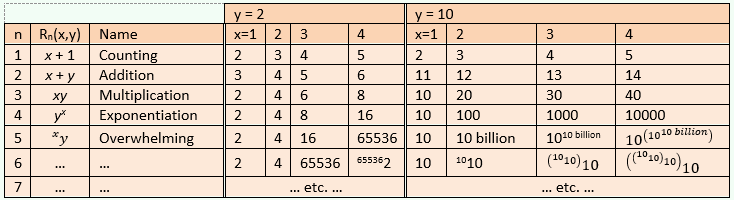10 billion is a 1 with 10 zeros.
1010 billion is a 1 with 10 billion zeros.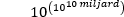is a 1 followed by 1010 billion zeros.
1010 is unimaginably much larger.
And so on ... Isn't it fun?

Googol, Skewes, Knuth, Ackermann
There are some famous numbers in mathematics. One of them is 1 googol (not: google, that's something else). 1 googol is defined as 10100. That number is in the table in row 5, at y=10, somewhere between x=2 and x=3.

Then there is also 1 googolplex. That is defined as 10googol. This value is also in the table in row 5, at y=10, but a bit to the right: somewhere between x=3 and x=4.

Next, there is the number of Skewes, or actually several versions of Skewes. One of them is: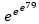. This number is also in the table in row 5 at y=10, somewhere to the right of x=4 but to the left of the (not shown) x=5.

At the moment I'm typing this it's not 1982 anymore. It's way past 2000, and I can google and wiki as much as I want on subjects like this. This is how I discover in the English Wikipedia: Knuth's up-arrow notation from 1976. That corresponds well with what I've come up with. Older is the Ackermann function from 1928.

Both are of course older than my idea from 1982, and that doesn't surprise me: as I wrote above, it seems very unlikely to me that, as a mathematics amateur, I can add something really original to mathematics: I don't expect that at all. Again: the fun is in the solving. Not the originality.